# Lagrange, Euler, and Kovalevskaya tops facts for kids

Kids Encyclopedia Facts

In classical mechanics, the precession of a rigid body such as a top under the influence of gravity is not, in general, an integrable problem. There are however three (or four) famous cases that are integrable, the Euler, the Lagrange, and the Kovalevskaya top. In addition to the energy, each of these tops involves three additional constants of motion that give rise to the integrability.

The Euler top describes a free top without any particular symmetry, moving in the absence of any external torque in which the fixed point is the center of gravity. The Lagrange top is a symmetric top, in which two moments of inertia are the same and the center of gravity lies on the symmetry axis. The Kovalevskaya top is a special symmetric top with a unique ratio of the moments of inertia which satisfy the relation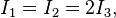$I_1=I_2= 2 I_3,$

That is, two moments of inertia are equal, the third is half as large, and the center of gravity is located in the plane perpendicular to the symmetry axis (parallel to the plane of the two equal points). The nonholonomic Goryachev–Chaplygin top (introduced by D. Goryachev in 1900 and integrated by Sergey Chaplygin in 1948) is also integrable (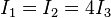$I_1=I_2=4I_3$). Its center of gravity lies in the equatorial plane. It has been proven that no other holonomic integrable tops exist .

## Hamiltonian formulation of classical tops

A classical top is defined by three principal axes, defined by the three orthogonal vectors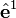$\hat{\mathbf{e}}^1$,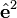$\hat {\mathbf{e}}^2$ and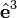$\hat{\mathbf{e}}^3$ with corresponding moments of inertia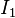$I_1$,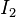$I_2$ and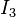$I_3$. In a Hamiltonian formulation of classical tops, the conjugate dynamical variables are the components of the angular momentum vector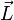$\vec{L}$ along the principal axes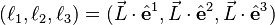$(\ell_1, \ell_2, \ell_3)= (\vec{L}\cdot \hat {\mathbf{e}}^1,\vec{L}\cdot \hat {\mathbf{e}}^2,\vec{L}\cdot \hat {\mathbf{e}}^3)$

and the z-components of the three principal axes,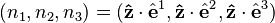$(n_1, n_2, n_3)= (\mathbf{\hat{z}}\cdot \hat {\mathbf{e}}^1,\mathbf{\hat{z}}\cdot \hat {\mathbf{e}}^2,\mathbf{\hat{z}}\cdot \hat {\mathbf{e}}^3)$

The Poisson algebra of these variables is given by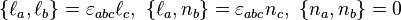$\{ \ell_a, \ell_b\} = \varepsilon_{abc} \ell_c, \ \{\ell_a, n_b\} = \varepsilon_{abc} n_c, \ \{n_a, n_b\} = 0$

If the position of the center of mass is given by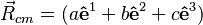$\vec{R}_{cm} = (a \mathbf{\hat e}^1 + b \mathbf{\hat e}^2 + c\mathbf{\hat e}^3)$, then the Hamiltonian of a top is given by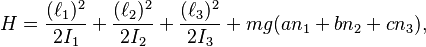$H = \frac{(\ell_1)^2}{2I_1}+\frac{(\ell_2)^2}{2I_2}+\frac{(\ell_3)^2}{2I_3}+ mg (a n_1 + bn_2 + cn_3),$

The equations of motion are then determined by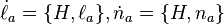$\dot{\ell}_a = \{ H, \ell_a\}, \dot{n}_a = \{H, n_a\}$

## Euler top

The Euler top is an untorqued top, with Hamiltonian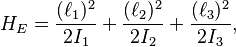$H_E = \frac{(\ell_1)^2}{2I_1}+\frac{(\ell_2)^2}{2I_2}+\frac{(\ell_3)^2}{2I_3},$

The four constants of motion are the energy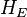$H_E$ and the three components of angular momentum in the lab frame,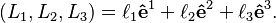$(L_1,L_2,L_3) = \ell_1 \mathbf{\hat e}^1 +\ell_2\mathbf{\hat e}^2+ \ell_3 \mathbf{\hat e}^3.$

## Lagrange top

The Lagrange top (so named after Joseph-Louis Lagrange) is a symmetric top with the center of mass along the symmetry axis at location,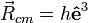$\vec{R}_{cm} = h\mathbf{\hat e}^3$, with Hamiltonian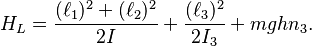$H_L= \frac{(\ell_1)^2+(\ell_2)^2}{2I}+\frac{(\ell_3)^2}{2I_3}+ mgh n_3.$

The four constants of motion are the energy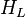$H_L$, the angular momentum component along the symmetry axis,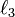$\ell_3$, the angular momentum in the z-direction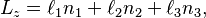$L_z = \ell_1n_1+\ell_2n_2+\ell_3n_3,$

and the magnitude of the n-vector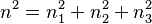$n^2 = n_1^2 + n_2^2 + n_3^2$

## Kovalevskaya top

The Kovalevskaya top is a symmetric top in which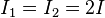$I_1=I_2=2I$,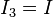$I_3=I$ and the center of mass lies in the plane perpendicular to the symmetry axis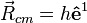$\vec R_{cm} = h \mathbf{\hat e}^1$. It was discovered by Sofia Kovalevskaya in 1888 and presented in her paper "Sur le problème de la rotation d'un corps solide autour d'un point fixe", which won the Prix Bordin from the French Academy of Sciences in 1888. The Hamiltonian is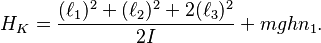$H_K= \frac{(\ell_1)^2+(\ell_2)^2+ 2 (\ell_3)^2}{2I}+ mgh n_1.$

The four constants of motion are the energy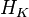$H_K$, the Kovalevskaya invariant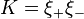$K = \xi_+ \xi_-$

where the variables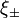$\xi_\pm$ are defined by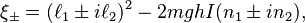$\xi_\pm = (\ell_1\pm i \ell_2 )^2- 2 mgh I(n_1\pm i n_2),$

the angular momentum component in the z-direction,$L_z = \ell_1n_1+\ell_2n_2+\ell_3n_3,$

and the magnitude of the n-vector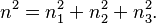$n^2 = n_1^2 + n_2^2 + n_3^2.$Lagrange, Euler, and Kovalevskaya tops Facts for Kids. Kiddle Encyclopedia.